Next: Solution to the Hamilton-Jacobi Up: Laser-driven particle mechanics Previous: The Dynamical Phase   Contents

# The Hamilton-Jacobi Equation for a Relativistic Particle.

Being defined in terms of the action integral, the dynamical phase satisfies a differential equation which one obtains by a simple argument:

Let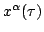and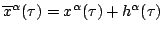be two worldlines having the same starting pointboth satisfying Lagrange's equation of motion, but having slightly different termination points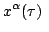andas in Figure 4. Then the (principal linear part of the) difference in the value of the dynamical phase at these termination points is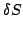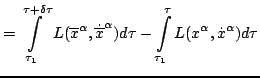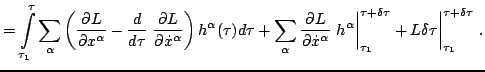The fact thatsatisfies Lagrange's equation of motion implies that the integral vanishes. Recalling the definition of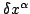, or looking at Figure 4, one sees that at the two termination points one hasConsequently, the principal linear part of the difference between the twovalues at the termination point is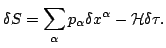(10)

Here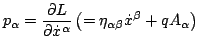are the momentum components and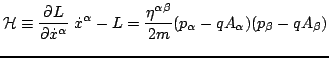is the superhamiltonian of the charged particle at the termination point of its worldline. Equation (11) is the expression for the differential of. One has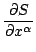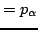Thus the differential equation for the dynamical phase functionis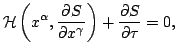(11)

or explicitlyThis is the Hamilton-Jacobi for a charged particle in an electromagnetic vector potential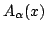.Next: Solution to the Hamilton-Jacobi Up: Laser-driven particle mechanics Previous: The Dynamical Phase   Contents
Ulrich Gerlach 2005-11-07